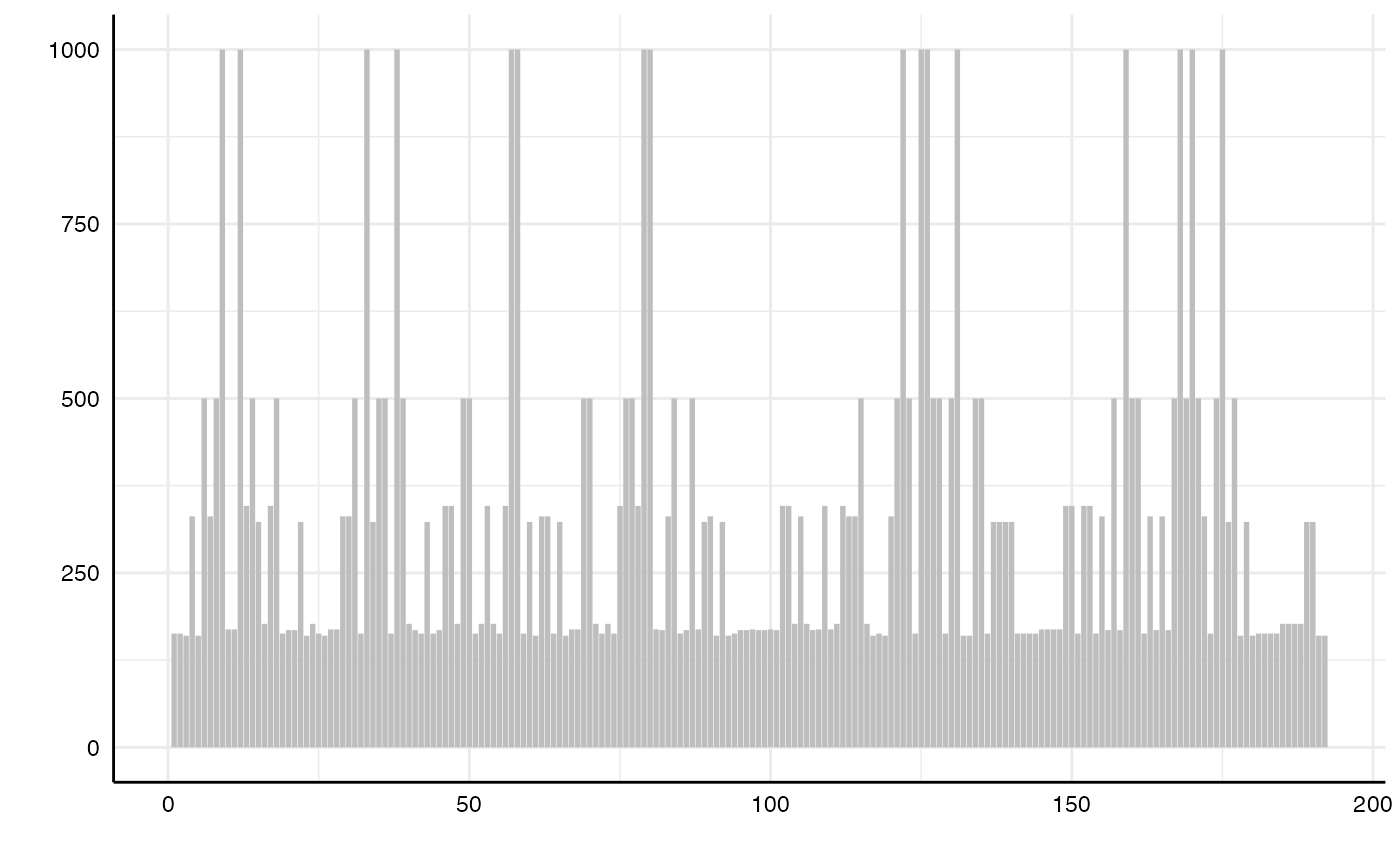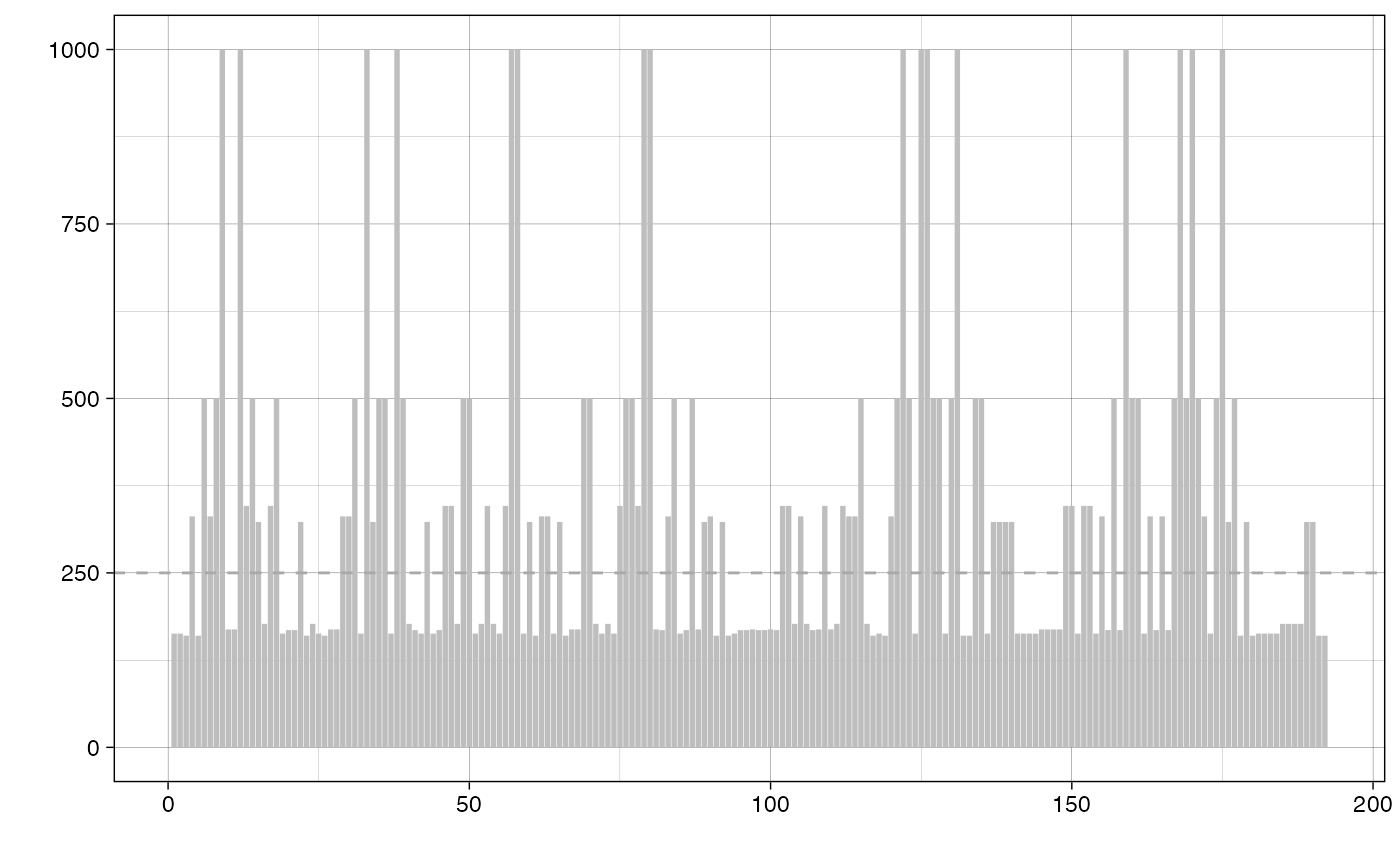This function plots a histogram of sample sizes per specification. It can be added to the overall specification curve plot (see vignettes).

plot_samplesizes(df, var = .data$estimate, desc = FALSE) ## Arguments df a data frame resulting from run_specs(). var which variable should be evaluated? Defaults to estimate (the effect sizes computed by run_specs()). desc logical value indicating whether the curve should the arranged in a descending order. Defaults to FALSE. ## Value a ggplot object. ## Examples # load additional library library(ggplot2) # for further customization of the plots # run specification curve analysis results <- run_specs(df = example_data, y = c("y1", "y2"), x = c("x1", "x2"), model = c("lm"), controls = c("c1", "c2"), subsets = list(group1 = unique(example_data$group1),
group2 = unique(example_data$group2))) # plot ranked bar chart of sample sizes plot_samplesizes(results)# add a horizontal line for the median sample size plot_samplesizes(results) + geom_hline(yintercept = median(results$fit_nobs),
color = "darkgrey",
linetype = "dashed") +
theme_linedraw()